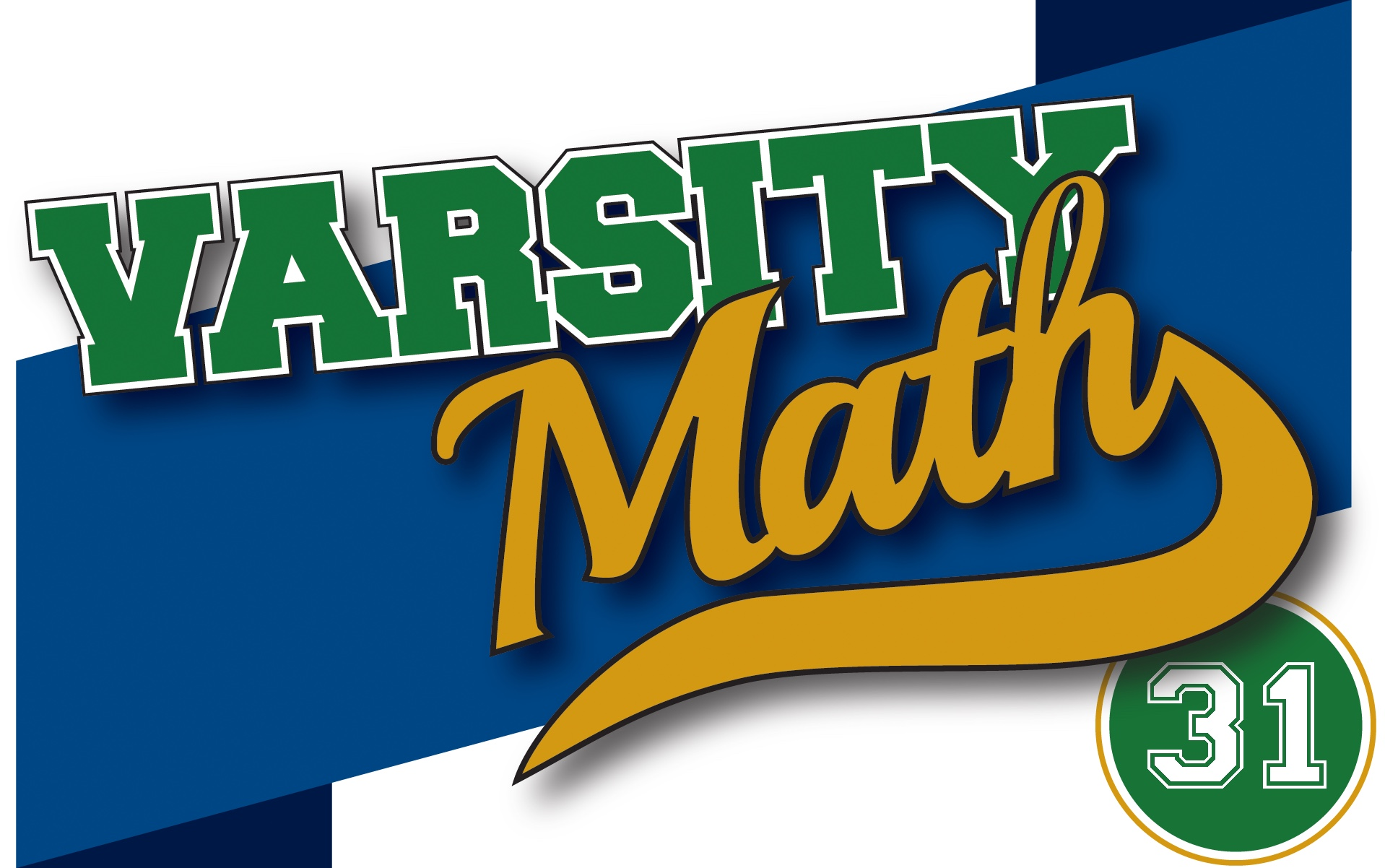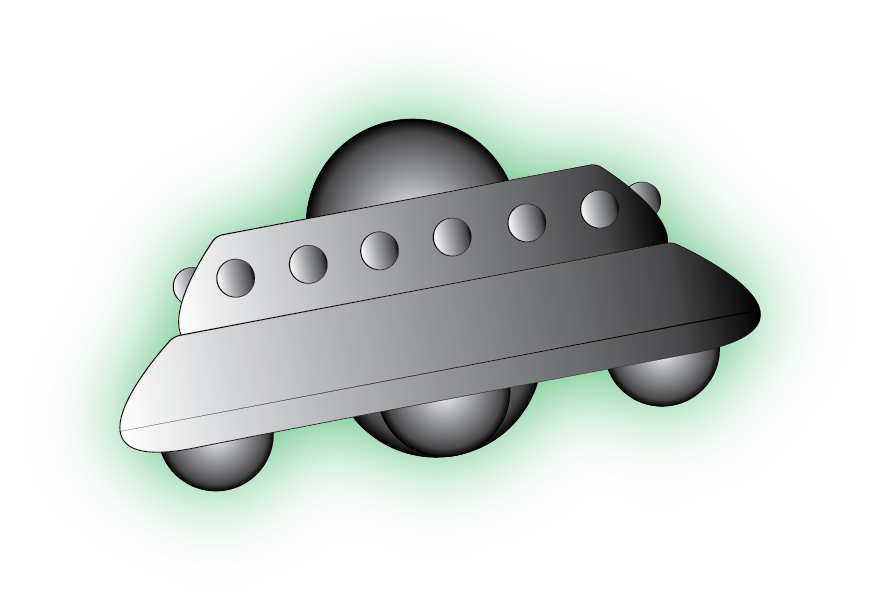Apparently the team members have been concentrating really hard on practices, because now math puzzles are starting to show up in their sleep…

### Alien ArithmeticOne Varsity Math team member dreams about his niece and nephew Polly and Sam. In the dream, an alien flies down to Earth and confronts the two kids, saying: “I have chosen two different numbers between 3 and 121, inclusive.” The alien then whispers the sum of the two numbers to Sam, and their product to Polly. “Now, Earth children, what can you tell me about my numbers?” Polly and Sam take after their uncle in their mathematical prowess, and Sam quickly observes, “I know Polly can’t tell what the two numbers are.” Polly then chimes in, “Well, that was true, but now I do know the two numbers!” And Sam concludes, “Oh, then I know the numbers, too!” The alien seems to smile, says “I guess Earth is a civilized planet after all!” and flies off as the dream ends. Shortly after waking, the team member realizes he knows the numbers as well.

What are the alien’s two numbers? Coach Newton would like to thank Professor (and Mathemagician) Art Benjamin of Harvey Mudd College for providing this puzzle. [Note that there was an error when this problem was originally published; the upper limit on the alien’s numbers was incorrectly listed as 97.]

### Dream Sequence

Sharene dreams that she is in an exam room with blue walls, and the examiner is droning on: “The number you are required to calculate is the square root of another quantity. And that quantity is basically one, so this should be an easy problem. But it’s not exactly one; you first have to add another number to one before you take the square root. To find out what number you add to one, proceed to the next room down the hall.” In the next room, with green walls: “The number you are required to add is basically the answer to last week’s Split Vote puzzle. But not quite. You must first multiply that number by the number you are required to calculate in the next room down the hall.” That third room has blue walls again, and the examiner says exactly the same thing as in the first room! The fourth room has green walls, and the examiner says the same thing as in the second room, except that this time, the number he mentions is one larger than the answer to last week’s Split Vote puzzle. The rooms follow one after the other, with every blue room identical and every green room mentioning a number one larger than the last. And then Sharene sees that there are infinitely many rooms proceeding down the hallway. As she is about to succumb to despair, she awakes and suddenly has a flash of insight: it is possible to compute the number required in the first room without visiting the infinite rooms beyond (assuming that the pattern of numbers mentioned continues indefinitely).

What is the number required to calculate in the first room of Sharene’s dream?

## Solution to Week 30

Treasure Trek. Imagine the three treasures buried anywhere in the square mile. If you move any one directly away from the center of the circle that the three of them lie on, then all of the distances between them become greater, and you can do this until each treasure has hit the boundary of the square. So we know that in the best places to bury them, the treasures are all on the boundary. Moreover, once this has happened, consider the diagonal of the square. If all three are on one side of the diagonal, then flipping the one closest to the diagonal to its mirror-image position in the diagonal will only increase the pairwise distances, so we can assume that there is one on one side of the diagonal, and two on the other side of the diagonal. Now, we can move the one that’s alone in its half to the corner farthest from the diagonal, only increasing the pairwise distances.

Thus, we have shown that the optimal configuration must consist of one treasure at a corner of the square and the other two on the two far sides. (They must be on different sides because we could otherwise improve things by flipping one of them over the other diagonal of the square.) So let the coordinates of the three points, in miles, be (0,0), (x, 1), and (1,y). There are two possibilities: (A) the side from (0,0) to (x, 1) is the shortest side, or (B) it is not the shortest side. In the first case, it is clear that the length of the shortest path among the treasures is obtained when the triangle is isosceles (since otherwise we could lengthen that path by making the shorter of the other two sides a bit longer). That occurs when the distance from (x, 1) to (1,y) equals the distance from (0,0) to (1,y), or in equations, using the more convenient squares of the distances, when (1-x)² + (1-y)² = y² + 1, i.e. y = (1-x)²/2. This case holds as long as the distance from (0,0) to (x, 1) is less than or equal to the common length of the other two sides, i.e. until x is large enough that the inscribed triangle is equilateral. That occurs when x² + 1 = (1-x)⁴/4 + 1, at x = 2 – √3. What about case (B)? This is completely symmetric to the first case, with the roles of x and y interchanged, so we need only consider case A and x in the closed interval from 0 to 2 – √3.

In particular, we need to find the x in this interval that makes the total distance to dig up all three treasures, namely √((1-x)⁴/4 + 1) + √(x² + 1), as large as possible. There are many techniques to find that maximum, but Coach Newton always wants us to try to solve the problems without calculus, so notice that the first term √((1-x)⁴/4 + 1) is steadily decreasing and concave up as x goes from 0 to 1, and the second term √(x² + 1) is steadily increasing and concave up on the same interval, and hence their sum can only change direction from increasing to decreasing or vice versa once on that interval. So to find the maximum, we try the endpoints and a point in the middle of the interval. At x = 0, the path length is √5/2 + 1; at x = 2 – √3, the path length is √(8-4√3) + √(8-4√3) = 4√(2-√3), and at x = 1/4, the path length is √(1105/1024) + √(17/16). The first of these is larger than 2.11, the second is just over 2.7, and the last is just under 2.7. Since the one in the middle is less than either endpoint, the maximum on the interval occurs at the left-hand endpoint. In other words, the farthest that Prospector Pete can force you to walk to dig up all three treasures occurs by burying the treasures at the corners of the equilateral triangle with vertices at (0,0), (1,0), and (1,1/2), and is √5/2 + 1 miles — between 2.11 and 2.12 miles.

## Recent Weeks

Links to all of the puzzles and solutions are on the Complete Varsity Math page.

Come back next week for answers and more puzzles.